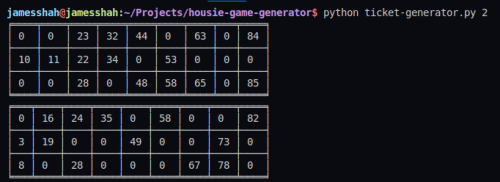# Housie(Tambola) Ticket Generator using Python

Housie aka Tambola aka Bingo (British Version) is probably the best game to play in Lockdown to get our minds off from Corona and all the negative news from all around the world and to have fun with friends and family. I've been playing it a lot,lately. For those who don't know what Housie is, let me explain.

# What is Housie?

Housie is basically a game of probability in which players mark off numbers on cards as the numbers are drawn randomly by a caller. So at the beginning of the game, all the players gets a ticket [3x9 matrix] in which there are total 27 boxes and 15 of them are filled with a distinct random number from 1 to 90. Here is a sample housie ticket.And then a caller calls random number from 1 to 90 and the winner being the first person to mark off all their numbers. So, It's pretty simple yet interesting and gripping game that one can play with friends and family.

# Housie Ticket Rules

Now there are some rules to generate housie tickets and you can't just randomly put 15 numbers in any boxes of your choice. So, let's see the rules and then create a Python script that will generate such housie ticket for us.

RULE #1 - Each row cannot have more than 5 numbers.

RULE #2 - Each column is assigned a range of numbers only:
(ex. 1-10 can appear only in column 1)

RULE #3 - In a specific column, numbers must be arranged in ascending order from top to bottom.

Now, ofcourse it's a cumbersome task(and a little biased,too) for a human to generate such tickets, and for the record we are talking about atleast 20-25 tickets per game. So yeah it's definitely not a fun task. So, I thought of creating a python script that would take the number of tickets that we want to generate as input and will return the tickets as an output.

I know, it's not at all a very fancy or difficut script to write but anyway I am free in this lockdown period so I thought why not?

Let's see how we are gonna build this script.

### Step 1 : Create a 3x9 matrix (array) of zeros

``````import numpy as np

# Create a 2D array[3x9] of 0s
ticket_array = np.zeros((3, 9), dtype=int)``````

Here I've used `numpy` library of Python to create the `ticket_array` because while creating a single matrix, it may not be effective but when we want to create very large number of lists, numpy creates it very fast and therefore it is efficient to use numpy to create the array.

### Step 2: Randomly Generate 15 indices to fill values in a ticket.

Now, in numpy we can use tuple for indexing of an array. i.e. To get the first element of first row and first column from `ticket_array` , we can use `ticket_array[(0,0)]`.

And so, first we'll generate an array `total_indices` which contains all the indices of 3x9 array as tuples (i.e. (0,0), (0,1)), (0,2), ..., (2,8))

and then, we'll randomly generate 5 indices from each row.

``````total_indices = [(i, j) for i in range(3) for j in range(9)]

random_indices = []

first_row = random.sample(total_indices[:9], 5)
second_row = random.sample(total_indices[9:18], 5)
third_row = random.sample(total_indices[-9:], 5)

for i in first_row:
random_indices.append(i)

for i in second_row:
random_indices.append(i)

for i in third_row:
random_indices.append(i)``````

### Step 3: Now Let's fill this indices with random values

So let's create a list ranging from 1 to 90. Then to satisfy the Rule #2, we'll check the column number from index and will fill the according value.

``````for num in random_indices:
if num == 0:
number = random.choice(total_numbers[:10])
ticket_array[num] = number
total_numbers[total_numbers.index(number)] = 0
elif num == 1:
number = random.choice(total_numbers[10:20])
ticket_array[num] = number
total_numbers[total_numbers.index(number)] = 0
elif num == 2:
number = random.choice(total_numbers[20:30])
ticket_array[num] = number
total_numbers[total_numbers.index(number)] = 0
elif num == 3:
number = random.choice(total_numbers[30:40])
ticket_array[num] = number
total_numbers[total_numbers.index(number)] = 0
elif num == 4:
number = random.choice(total_numbers[40:50])
ticket_array[num] = number
total_numbers[total_numbers.index(number)] = 0
elif num == 5:
number = random.choice(total_numbers[50:60])
ticket_array[num] = number
total_numbers[total_numbers.index(number)] = 0
elif num == 6:
number = random.choice(total_numbers[60:70])
ticket_array[num] = number
total_numbers[total_numbers.index(number)] = 0
elif num == 7:
number = random.choice(total_numbers[70:80])
ticket_array[num] = number
total_numbers[total_numbers.index(number)] = 0
elif num == 8:
number = random.choice(total_numbers[80:89])
ticket_array[num] = number
total_numbers[total_numbers.index(number)] = 0``````

### Step 4: Now let's sort the ticket_array column wise

``````for col in range(9):

# if all the rows are filled with random number

if(ticket_array[col] != 0 and ticket_array[col] != 0 and ticket_array[col] != 0):
for row in range(2):
if ticket_array[row][col] > ticket_array[row+1][col]:
temp = ticket_array[row][col]
ticket_array[row][col] = ticket_array[row+1][col]
ticket_array[row+1][col] = temp

# if 1st and 2nd row are filled by random number

elif(ticket_array[col] != 0 and ticket_array[col] != 0 and ticket_array[col] == 0):
if ticket_array[col] > ticket_array[col]:
temp = ticket_array[col]
ticket_array[col] = ticket_array[col]
ticket_array[col] = temp

# if 1st and 3rd row are filled by random number

elif(ticket_array[col] != 0 and ticket_array[col] != 0 and ticket_array[col] == 0):
if ticket_array[col] > ticket_array[col]:
temp = ticket_array[col]
ticket_array[col] = ticket_array[col]
ticket_array[col] = temp

# if 2nd and 3rd rows are filled with random numbers

elif(ticket_array[col] == 0 and ticket_array[col] != 0 and ticket_array[col] != 0):
if ticket_array[col] > ticket_array[col]:
temp = ticket_array[col]
ticket_array[col] = ticket_array[col]
ticket_array[col] = temp``````

### Step 5: Wrap it up in a function and print the tickets.

To print the tickets I used tabulate package of python using which we can present array and matrix in a neat way. Learn more about Tabulate Here.

``````if __name__ == "__main__":

# Take number of tickets from user as system argument
numberOfTickets = sys.argv
tickets = []

for i in range(int(numberOfTickets)):
ticket = getTickets()
tickets.append(ticket)

for ticket in tickets:
print(tabulate(ticket, tablefmt="fancy_grid", numalign="center"))``````

And Done!! Now, Let's check by running the script from terminal.

``\$ python ticket-generator.py 2 ``

Output:You can checkout the full code on Github : Housie Ticket Generator